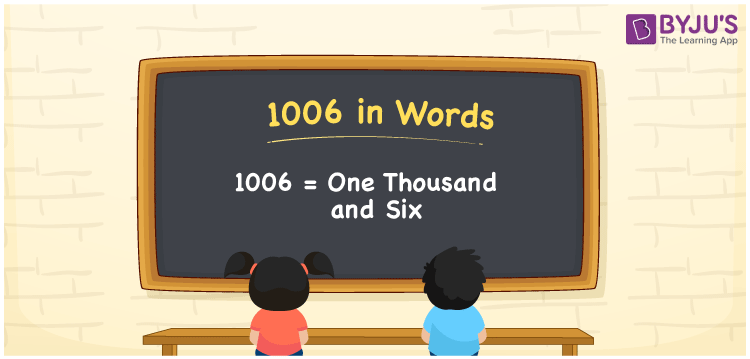# 1006 in Words

We can write 1006 in words as One thousand and six. We can effortlessly convert the number 1006 into words with the help of a place value chart. If you paid an Rs. 1006 electricity bill, you can say, “I paid an electricity bill of One thousand and six rupees”. In this article, you can learn how to convert the cardinal number 1006 into words.

 1006 in words One thousand and six One thousand and six in numerical form 1006

## 1006 in English Words

We generally write numbers in words using the English alphabet. Thus, we can spell 1006 in English as “One thousand and six”.## How to Write 1006 in Words?

Let us create a four-column place value chart to write the number 1006 in words since it has four digits. This is illustrated in the table below.

 Thousands Hundreds Tens Ones 1 0 0 6

Here, ones = 6, tens = 0, hundreds = 0, thousands = 1

Thus, we can show the expanded form of 1006 using the above place values and digits as:

1 × Thousand + 0 × Hundred + 0 × Ten + 6 × One

= 1 × 1000 + 0 × 100 + 0 × 10 + 6 × 1

= 1000 + 6

= One thousand + Six

= One thousand and six

Therefore, 1006 in words = One thousand and six.

As we know, 1006 is a natural number that precedes 1007 and succeeds 1005.

1006 in words – One thousand and six

Is 1006 an odd number? – No

Is 1006 an even number? – Yes

Is 1006 a perfect square number? – No

Is 1006 a perfect cube number? – No

Is 1006 a prime number? – No

Is 1006 a composite number? – Yes

## Frequently Asked Questions on 1006 in Words

Q1

### How do you write 1006 in words?

Generally, we write 1006 in English words as One thousand and six.
Q2

### How to write Rs. 1006 in words on a cheque?

On a cheque, we can write 1006 in words as One thousand and six rupees only.
Q3

### What is the number name of 1006?

One thousand and six is the number name of 1006.
Test your Knowledge on 1006 in Words